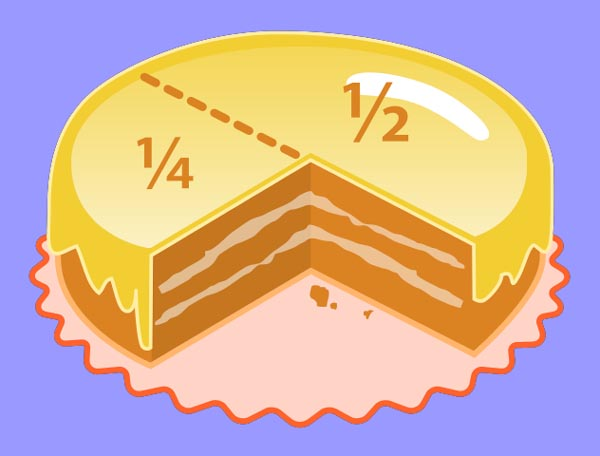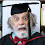## March 12, 2012Fractions of a cake(Wikipedia)

Note: What are numerators and denominators? How do we keep them straight? And how do we pronounce fractions in English? The answers are here!

Get Ready: Do you have a good understanding of fractions? When did you first learn about them?

Becky is chatting with her classmate Lily in the Common Room of their dorm.

Becky: Hey, Lily.

Lily: Hey, Becks. Can you help me with math for a minute?

Becky: Math? I thought you were a whiz at that!

Lily: I guess I am, sorta. But it's talking about this stuff in English that's got me going around in circles.

Becky: Now, that I understand. So, what's the problem?

Lily: Fractions.

Becky: Ugh. Nobody loves fractions. So?

Lily: First, what do you call the top part and the bottom part?

Becky: The top part, like the "one" in one-half, is called the numerator. It tells the number of parts. Get it?

Lily: Yeah. And the bottom half?

Becky: The denominator. Nom- is a root meaning "name"; the denominator names the type of parts, like halves, thirds, quarters, and so on.

Lily: Got it! My teacher keeps talking about "least common denominators."

Becky: Least whaha?

Lily: When you add or subtract two fractions with different denominators, you have to find a denominator both can share.

Becky: Uh. Huh.

Lily: Like to add 1/3 and 1/6, you can use...

Becky: Eighteen!

Lily: Well, you can, but that's not the least. Actually, six is the LCD of both three and six.

Becky: Oh. Sooo... we change 1/3 to 2/6, and 2/6 + 1/6 = 3/6. Right?

Lily: Yes, but now you have to reduce that fraction.

Becky: Reduce it to what?

Lily: Well, 3/6 is the same as 1/2, so that's the best answer.

Becky: Wow, you really are a whiz!

Lily: C'mon, Becky; this is kid's stuff.

Becky: Not for me! By the way, let me tell you one more thing: when we say the numerator, we use "counting numbers": one, two, three, etc.

Lily: Right.

Becky: And for denominators, we use "ordinal numbers": third, fourth, fifth, etc., plus their plurals, like two thirds, three quarters, etc.

Lily: Isn't there something special about denominators of two and four?

Becky: Well, we never say seconds. That would be "half" or "halves." And we while can say fourths, we might also say quarters, like I just did: three quarters.

Lily: Wow! Thanks for teaching me English, Becky!

Becky: Thanks for teaching me math, Lily!

NOTE: Here are some pronunciation examples: 1/2 = one half; 3/2 = three halves; 2/3 = two thirds; 3/4 = three fourths or three quarters; 1/5 = one fifth; 5/6 = five sixths; 6/7 = six sevenths; 1/8 = one eighth; 7/9 = seven ninths

--------

Practice: Match the term to its definition or pronunciation below:

1. 1/2
2. 1/3
3. 1/6
4. 2/6 + 1/6 = 3/6
5. denominator
6. fractions
7. least common denominator
8. Least whaha?
9. Now, THAT I understand
10. numerator
11. reduce a fraction
12. this is kid's stuff
13. going around in circles
14. Uh. Huh.
15. whiz

1. pronounced "two sixths plus one sixth equals three sixths."
2. I understand that; it can be used with different verbs.
3. pronounced "one sixth"
4. the "2" in 2/3; the top of the fraction. It tells the number of parts.
5. pronounced "one third"
6. someone who's good at something
7. also called LCD, it's the lowest denominator we can use to add or subtract fractions with different denominators
8. bring a fraction to its lowest terms, as in the example
9. numbers like 1/2, 2/3, etc. that describe part of something
10. the "3" in 3/5; the bottom of the fraction. It names the type of parts.
11. confused
12. pronounced "one half"
13. Becky isn't getting it, so says "uh-huh" (meaning "yes") slowly.
14. a joking question because she didn't understand something
15. This is easy

Answers are in the first comment below.

Submitted to the Shenzhen Daily for March 12, 2012

This lesson received 202 visits on my old site between March, 2012, and July, 2021.

#### 1 comment:

1.Answers to the Practice: 1. l; 2. e; 3. c; 4. a; 5. j; 6. i; 7. g; 8. n; 9. b; 10. d; 11. h; 12. o; 13. k; 14. m; 15. f